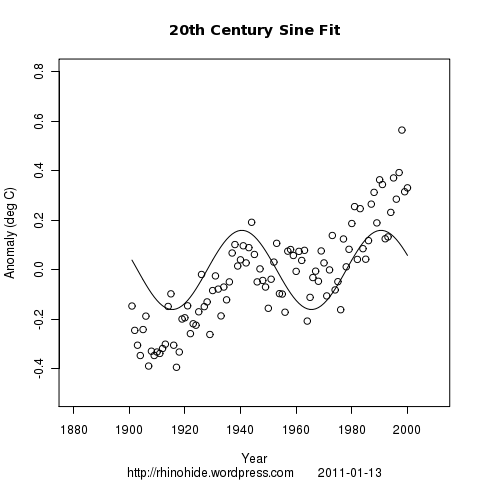Home > LSCF > Lines, Sines, and Curve Fitting 3 – double down

Lines, Sines, and Curve Fitting 3 – double down

2011 January 9

Today, I drop the linear fit, and fit two sine waves instead.

First, the sine wave on the data from 1900 to 2000Next we extract the residuals, the difference between the actual data points and the sine wave, and fit a sinusoidal trend.

The sine trend on the residuals looks like this:Now, just to add them together like this:How good is the fit?
The correlation of the sine to the data is 0.58.
The correlation of the sine to the residuals is 0.74.
And the correlation of the two sines to the original data is 0.86.

What are the parameters?
The amplitude (A) of the first sine is 0.16C.
The phase shift (b) is 28 years.
And the period (T) is 50 years.

The amplitude (A) of the second sine is 0.16C.
The phase shift (b) is 121 years.
And the period (T) is 141 years.

Interesting that the 2 sine waves have the same amplitude.

The script is here.

1. 2011 December 17 at 9:04 am

Data Transformation
Better half, this great site is without a doubt fabolous, i love it Data Transformation

1. 2011 February 13 at 10:41 am